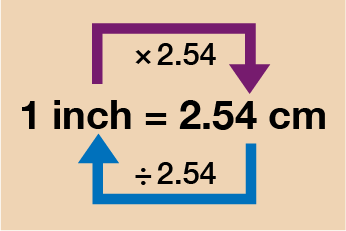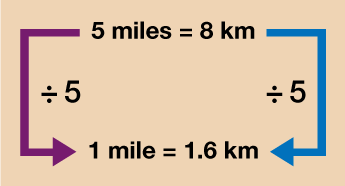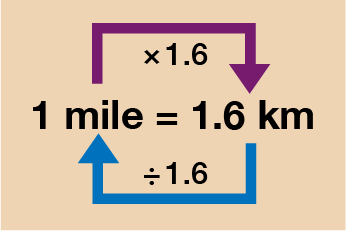Everyday maths 2 (Wales)

Start this free course now. Just create an account and sign in. Enrol and complete the course for a free statement of participation or digital badge if available.

Free course

# 2.2 Converting units of measure between different systems

This is a useful skill to have because you may often measure something in one unit, say your height in feet and inches, but then need to convert it to centimetres. You may, for example, be training to run a 10 km race and want to know how many miles that is. To do this all you need to know is the conversion rate and then you can use your multiplication and division skills to calculate the answer.

When performing these conversions, you are converting between metric and imperial measures. Metric measures are commonly used around the world but there are some countries (the USA for example) who still use imperial units. Whilst the UK uses metric units (g, kg, m, cm etc.) there are some instances where you may still need to convert a metric measure to an imperial one (inches, feet, gallons etc.).

In most cases, the conversion rate will be given in a similar format to the way money exchange rates are given, i.e. 1 inch = 2.5 cm or 1 kg = 2.2 lb. In these cases you can do exactly the same as you would with a currency conversion. The only exceptions here are where you don’t have one of the units of measure as a single unit i.e. 5 miles = 8 km.

As this skill is very similar to the one you previously learnt with currency conversions, let’s just look at two brief examples before you have a go at an activity for yourself.

## Example: Centimetres and inches

You want to start your own business making accessories. One of the items you will be making is tote bags. The material you have bought is 156 cm wide. You need to cut pieces of material that are 15 inches wide.

How many pieces can you cut from the material you bought?

Use 1 inch = 2.54 cm.

Method

You need to start by converting either cm to inches or inches to cm so that you are working with units in the same system. Let’s look at both ways so that you feel confident that you will get the same answer either way.Figure 10 Converting between inches and centimetres

You can see from the diagram above that to convert from inches to cm you must multiply by 2.54. To convert from cm to inches you must divide by 2.54.

As with currency conversions, if you do not have a diagram to help you, you will have to work out yourself whether you need to multiply by the conversion rate or divide by it. To do this, write down your conversion rate:

1 inch = 2.54 cm (remember to put the single unit – the 1 – on the left-hand side)

Converting from inches to cm

Now write the figure you are converting under the correct side:

1 inch = 2.54 cm

15 inches = ? cm

Since you want to know what 15 inches is in centimetres, you are going forwards so you need to multiply by the conversion rate of 2.54:

15 inches × 2.54 = 38.1 cm. This is the length of the material needed for each bag.

To calculate how many pieces of material you can cut you then do:

156 ÷ 38.1 = 4.0944…. As you need whole pieces of material, you need to round this answer down to 4 pieces.

Converting from cm to inches

You may have decided to convert from centimetres to inches instead. You still need to write down your conversion rate first:

1 inch = 2.54 cm (remember to put the single unit – the 1 – on the left-hand side)

Now write the figure you are converting under the correct side (in this case you will convert the 156 centimetres into inches):

1 inch = 2.54 cm

? inches = 156 cm

Since you want to know what 156 centimetres is in inches, you are going backwards so you need to divide by the conversion rate of 2.54:

156 ÷ 2.54 = 61.417…, this is the length of the big piece of material.

To calculate how many pieces of material you can cut you then do:

61.417… ÷ 15 = 4.0944…. Again, as you need whole pieces of material, you need to round this answer down to 4 pieces.

You can see that no matter which way you choose to convert you will arrive at the same answer.

Note: Some numbers do not divide into each other exactly so you end up with lots of digits after the decimal point. Where this is the case, you need to round off the answer. You may be told what to round off to in the question (e.g. to the nearest whole number or to two decimal places) or you may have to use your own judgement.

For money you will normally round to two decimal places. In this case you are thinking about whole pieces of material – you only have four whole pieces so this is what you round to here.

## Example: Kilometres and miles

You have signed up for a 60 km bike ride. There is a lake in a nearby park that you want to use for your training. You know that one lap of the lake is 2 miles. You want to cycle a distance of at least 40 km in your last training session before the race. How many full laps of the lake should you do?

You know that a rough conversion is 5 miles = 8 km.

There is more than one way to go about this calculation and, as ever, if you have a different method that works for you and you arrive at the same answer, feel free to use it!

Method

Since you already know how to convert if you are given a conversion that has a single unit of whichever measure you are using, it makes sense to just change the given conversion into one that you are used to dealing with.

Look at the diagram below – since you know that 5 miles is worth 8 km, you can find out what 1 mile is worth by simply dividing 5 by 5 so that you arrive at 1 mile. Whatever you do to one side, you must do to the other. Therefore, you also do 8 divided by 5. This then gives 1 mile = 1.6 km.Figure 11 Calculating 1 mile in kilometres

Now that you know 1 mile = 1.6 km, you can solve this problem in its usual way:Figure 12 Converting between miles and kilometres

1 mile = 1.6 km

Now write down the figure you want to convert under the correct side (in this case you want to convert 40 km into miles):

1 mile = 1.6 km

? miles = 40 km

Since you first want to know what 40 km is in miles, you are going backwards, so you need to divide by 1.6:

40 ÷ 1.6 = 25 miles

So, 40 km = 25 miles

You know that one lap of the lake is 2 miles so:

25 ÷ 2 = 12.5 laps

Therefore, you will need to cycle 12.5 laps around the lake in order to have cycled your target of 40 km.

Now that you have seen a couple of worked examples, have a go at the activity below to check your understanding.

## Activity 5: Converting between systems

Wherever possible, please do the calculations first without a calculator. You may then double-check on a calculator if needed.

1. A Ford Fiesta car can hold 42 litres of petrol. Using the fact that 1 gallon = 4.54 litres, work out how many gallons of petrol the car can hold. Give your answer rounded to two decimal places.
2. The café you work at has run out of milk and you have been asked to go to the shop and buy 10 pints. When you arrive however, the milk is only available in 2 litre bottles. You know that 1 litre = 1.75 pints. How many 2 litre bottles should you buy?
3. You are packing to go on holiday and are allowed 25 kg of luggage on the flight. You’ve weighed your case on the bathroom scales and it weighs 3 stone and 3 pounds.

You know that:

• 1 stone = 14 pounds
• 2.2 pounds = 1 kg

Is your luggage over the weight limit?

4. Your son wants to go on a ride at a theme park that has a minimum height restriction of 122 cm. You know your son is 4 ft. 7 in. tall. You know that:
• 1 foot (ft.) = 12 inches
• 1 inch (in.) = 2.54 cm

Can your child go on the ride?

1. You do:
• 1 gallon = 4.54 litres
• ? gallons = 42 litres
As you are converting litres to gallons, you are going backwards so need to divide by 4.54.
• 42 ÷ 4.54 = 9.25 gallons (to two d.p)
2. Firstly, you want to know how many litres there are in 10 pints, so you need to convert from pints to litres. You do:
• 1 litre = 1.75 pints
• ? litres = 10 pints

As you are converting pints to litres, you are going backwards so need to divide by 1.75:

• 10 ÷ 1.75 = 5.71… litres. As you can only buy the milk in 2 litre bottles, you will have to buy 6 litres of milk in total. You therefore need:
•  6 ÷ 2 = 3 bottles of milk
3. Firstly, you need to convert 3 stone and 3 pounds into just pounds. Since 1 stone = 14 pounds, to work out 3 stone you do:
• 3 × 14 = 42 pounds

Then you need to add on the extra 3 pounds, so in total you have 42 + 3 = 45 pounds.

Now that you know this, you can convert from pounds to kg.

Since 2.2 pounds = 1 kg, you can also say that 1 kg = 2.2 pounds (this doesn’t change anything, it just makes it a little easier as you can stick to your usual method of having the single unit on the left hand side):

• 1 kg = 2.2 pounds
• ? kg = 45 pounds

As you are converting from pounds to kg, you are going backwards so need to divide by 2.2:

• 45 ÷ 2.2 = 20.45 kg

Yes, your luggage is within the weight limit.

4. Firstly, you need to convert 4 ft. 7 in. into just feet. Since 1 foot = 12 inches, to work out 4 feet you do:
• 4 × 12 = 48 inches

Then you need to add on the extra 7 inches, so in total your son is 48 + 7 = 55 inches tall.

Now that you know this, you can convert from inches to centimetres:

• 1 inch = 2.54 cm
• 55 inches = ? cm

As you are converting from inches to centimetres, you are going forwards so need to multiply by 2.54:

• 55 × 2.54 = 139.7 cm

So your son is tall enough to go on the ride.

You should now be feeling confident with your conversion skills so it’s time to move on to the next part of this session.

## Summary

In this section you have learned:

• that different systems of measurement can be used to measure the same thing (e.g. a cake could be weighed in either grams or pounds)
• you can convert between these systems using your multiplication and division skills and the given conversion rate
• currencies can be converted in the same way as long as you know the exchange rate – this is particularly useful for holidays.
FSM_2_CYMRU#### Find out more

*Eligibility rules apply for financial support.